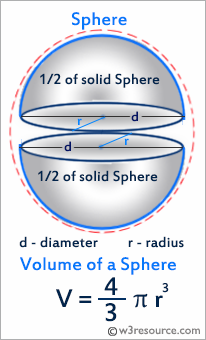# Python: Get the the volume of a sphere with radius 6

## Python Basic: Exercise-15 with Solution

Write a Python program to get the the volume of a sphere with radius 6.
Python: Volume of a Sphere
A sphere is a three-dimensional solid with no face, no edge, no base and no vertex. It is a round body with all points on its surface equidistant from the center. The volume of a sphere is measured in cubic units.
The volume of the sphere is : V = 4/3 × π × r3 = π × d3/6.Sample Solution :-

pi = 3.1415926535897931
r= 6.0
V= 4.0/3.0*pi* r**3
print('The volume of the sphere is: ',V)

=========== RESTART: F:/Python_APSC/py-ex-basic-15.py ===========
The volume of the sphere is:  904.7786842338603
>>>